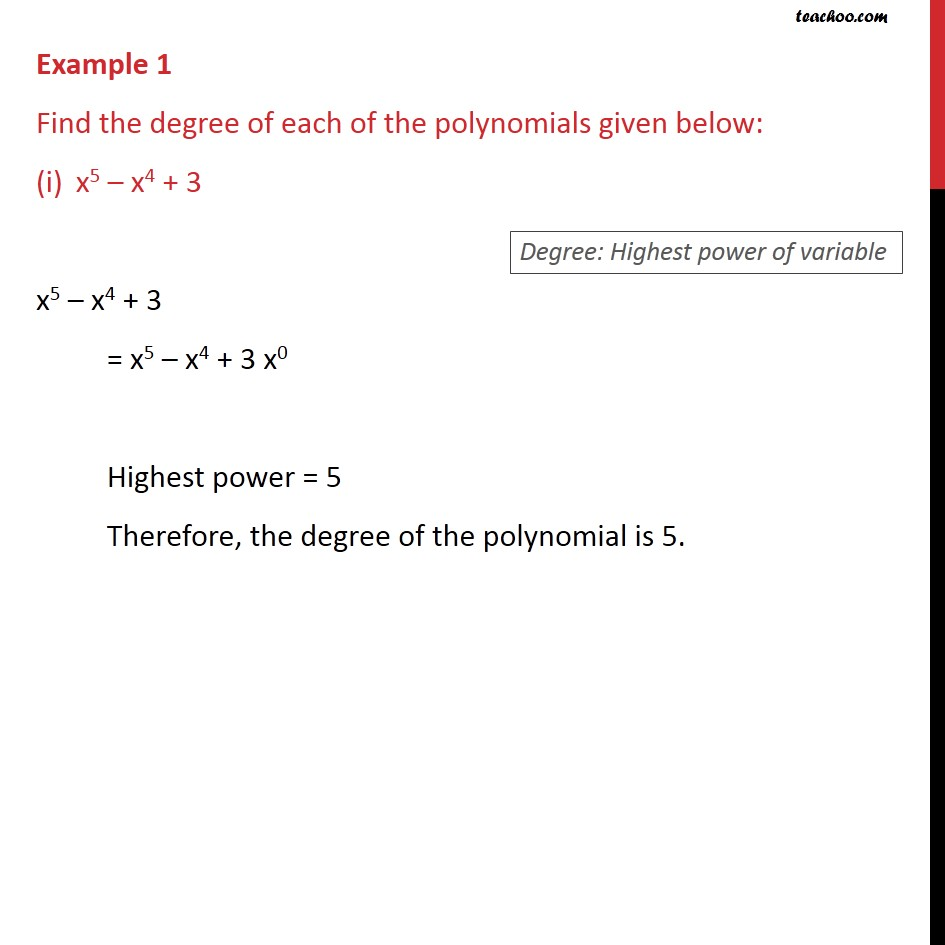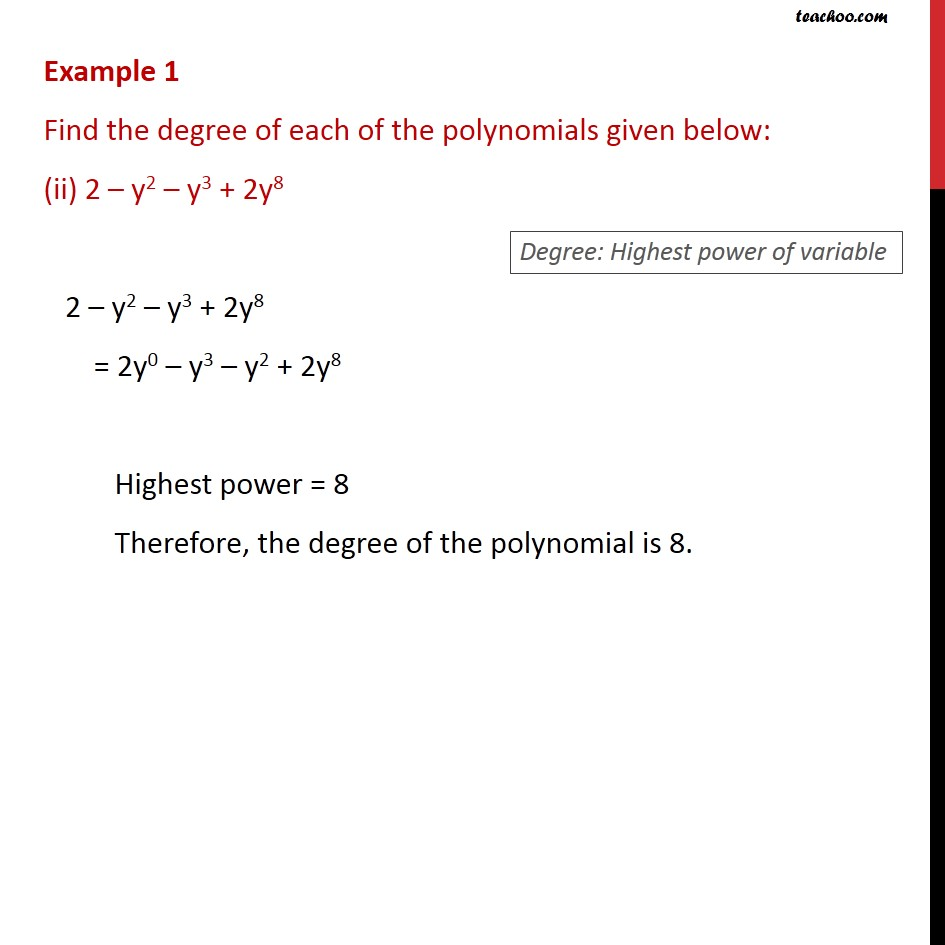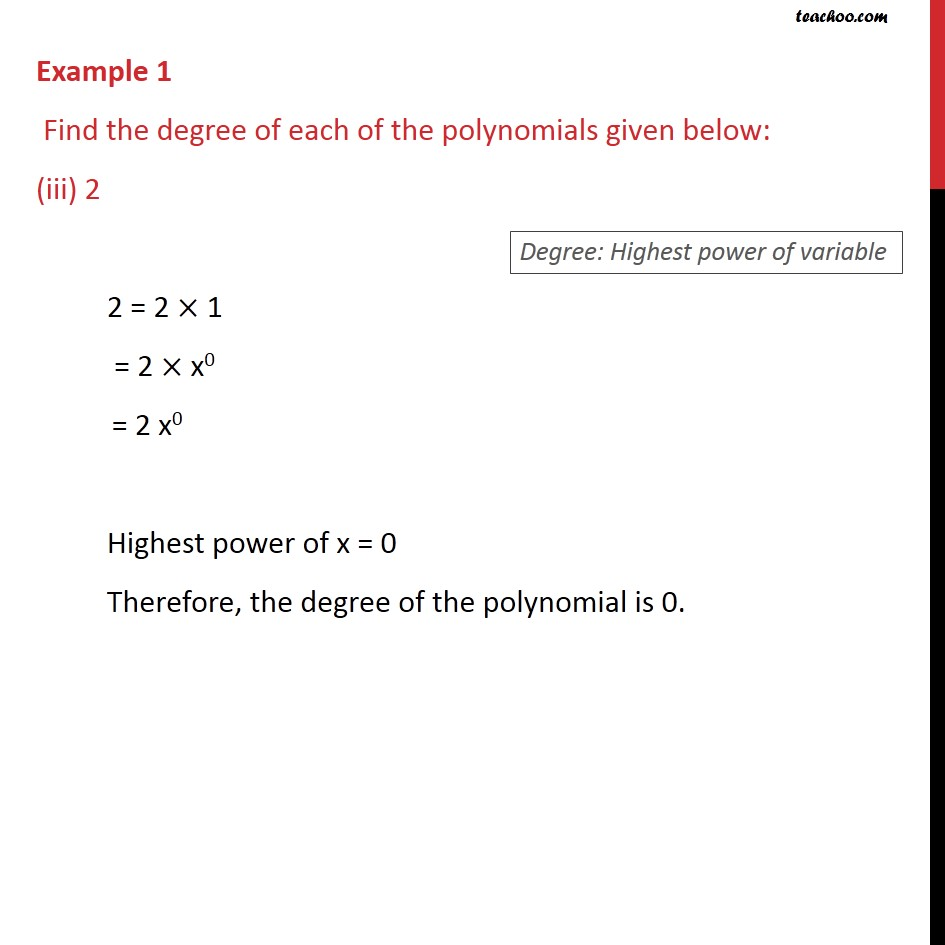Learn all Concepts of Polynomials Class 9 (with VIDEOS). Check - Polynomials Class 91. Chapter 2 Class 9 Polynomials
2. Concept wise
3. Degree & Coefficient of a polynomial

Transcript

Example 1 Find the degree of each of the polynomials given below: x5 x4 + 3 x5 x4 + 3 = x5 x4 + 3 x0 Highest power = 5 Therefore, the degree of the polynomial is 5. Example 1 Find the degree of each of the polynomials given below: (ii) 2 y2 y3 + 2y8 2 y2 y3 + 2y8 = 2y0 y3 y2 + 2y8 Highest power = 8 Therefore, the degree of the polynomial is 8. Example 1 Find the degree of each of the polynomials given below: (iii) 2 2 = 2 1 = 2 x0 = 2 x0 Highest power of x = 0 Therefore, the degree of the polynomial is 0.

Degree & Coefficient of a polynomial